#### Theory of Resistive Configurations and its applications

Introduction:

Resistors are the basic passive components of electric circuits. In high energy electric circuits they are employed to symbolize equivalent loads for power-dissipating processes or operations. In lower power electronic circuits, resistors are employed to produce voltages and currents at particular positions in a circuit to establish the accurate operating conditions for semiconductor devices. Therefore an understanding of the effect of differing configurations of resistor networks is necessary.

Resistors in Series:

Consider the configuration shown in figure below where N resistors are joined end-to-end and are termed to as being connected in series. A battery of emf E Volts is utilized to drive the circuit and hence a current I flows into the terminals of the combination of resistors. Note that the similar current flows via each of the resistors as charge should be conserved. The dashed line in the circuit is not intended to symbolize any electrical break in the connection however merely to point out that the series arrangement can be extended to any number (N) of resistors.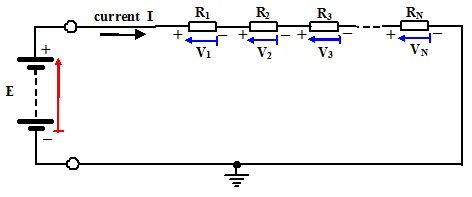Figure: A Configuration of Resistors Connected in Series

The man purpose is to find out the value of a single equivalent resistance, REQ, in such a way that if the series combination of resistors were substituted with this single value the circuit would behave in a same way in all respects. We wish for then a single resistance in such a way that in accordance with Ohm’s Law provides:

REQ = E/I

When the same current flows via each resistor then:

I = I1 = I2 = I3..........= IN

By Kirchhoff’s Voltage Law:

E1 - V1- V2- V3...... - VN = 0

Or,

E1 = V1 + V2 + V3.........+ VN

Then:

REQ = E/I = (V1 + V2 + V3.........+ VN)/I

REQ = E/I = V1/I + V2/I + V3/I +........... + VN/I

REQ = E/I = V1/I1 + V2/I2 + V3/I3 +........... + VN/IN

REQ = R1 + R2 + R3............+ RN

Thus it can be seen that the equal resistance is simply the sum of individual resistors.

Resistors in Parallel:

Consider the configuration shown in figure below where N resistors are joined side-by-side and are termed to as being connected in parallel. A battery of emf E Volts is employed to drive the circuit ad hence a current I flows into the terminals of the combination of resistors. Note that this time similar potential difference is developed across each of the resistors and is equivalent to the battery emf, E. The dashed line in the circuit is again not intended to symbolize any electrical break in the connection however just to point out that the parallel arrangement can be extended to any number of resistors, N.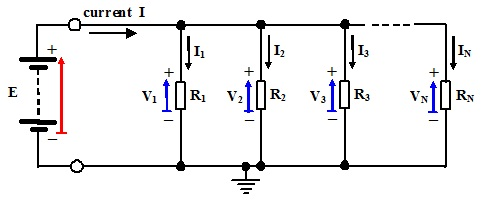Figure: A Configuration of Resistors Connected in Parallel

The main aim is to find out the value of a single equivalent resistance, REQ, in such a way that if the series parallel combination of resistors were substituted with this single value, the circuit would behave similar in all respects. We wish for a single resistance in such a way that in accordance with Ohm’s Law provides:

REQ = E/I

When same potential difference is developed across each resistor then:

E = V1 = V2 = V3.........= VN

By using Kirchhoff’s Current Law as applied to the top node of circuit:

I – I1 – I2 – I3........... –IN = 0

Or,

I = I1 + I2 + I3 ............. + IN

Then:

REQ = E/I = E/ (I1 + I2 + I3 ............. + IN)

Inverting provides:

I/REQ = (I1 + I2 + I3 ............. + IN)/E

I/REQ = I1/E + I2/E + I3/E ......... + IN/E

I/REQ = I1/V1 + I2/V2 + I3/V3 ......... + IN/VN

I/REQ = 1/R1 + 1/R2 + 1/R3.......... + 1/RN

This can as well be written somewhat cumbersomely as: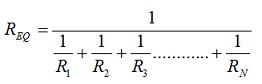Thus it can be seen that in the easiest form to express, the inverse of equivalent resistance is the sum of the inverses of individual resistors.

Consider the specific case of only two resistors in parallel:

I/REQ = 1/R1 + 1/R2 = (R1 + R2)/(R1R2) or REQ = (R1R2)/( R1 + R2)

This is sometimes termed to as the product over sum.

Applications:

Potential Divider:

In electronic circuits utilizing semiconductor and other devices as active elements is frequently essential to obtain a range of dc voltages other than the battery or supply voltage for the aims of biasing these devices for accurate operation or optimum performance. The simple potential divider network for this aim is shown in figure below where two resistors are joined is series across the battery supply.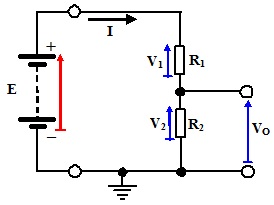Figure: A Potential Divider Network

The current I flow via both resistors and hence:

V0 = V2 = I2R2 = IR2

And,

I = E/REQ =E/(R1 + R2)

And hence:

V0 = [E/(R1 + R2)]R2 = [R2/( R1 + R2)]E

The ratio of resistors thus sets the potential VO as a fraction of the supply voltage E. Values of the resistors R1 and R2 can be selected accordingly to obtain any desired bias voltage from any supply voltage. This is of course possible to obtain a range of bias voltages by employing a chain of resistors joined in series across the supply.

Current Splitting:

At times in electronic circuits it essential to divide current among different paths or branches in a circuit. Out of the total current drawn from supply, it might be needed to deliver a fixed proportion or percentage to a specific element or load. A current splitting networks such as is shown in the figure below accomplishes this aim.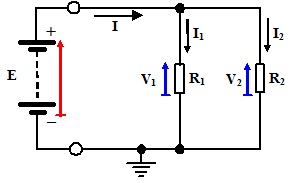Figure: A Current Splitting Network

The similar potential drop is developed across both resistors and hence:

V1 = V2 = E

And,

I = E/REQ = E/(R1|| R2) = [E (R1 + R2)]/(R1R2)  with,

I1 = V1/R1 = E/R1 and I2 = V2/R2 = E/R2

Then: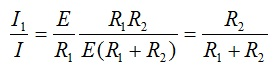That gives:

I1 = [R2/( R1 + R2)] I

Likewise: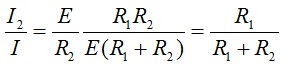That gives:

I2 = [R1/( R1 + R2)] I

Consider then: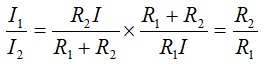This can be seen thus that the current drawn from the supply is dividing between the two branches of the circuit in the ratio of resistances of the opposite branches. This analysis can be taken out for a combination of more resistors in parallel however gives a more complex expression.

Latest technology based Electrical Engineering Online Tutoring Assistance

Tutors, at the www.tutorsglobe.com, take pledge to provide full satisfaction and assurance in Electrical Engineering help via online tutoring. Students are getting 100% satisfaction by online tutors across the globe. Here you can get homework help for Electrical Engineering, project ideas and tutorials. We provide email based Electrical Engineering help. You can join us to ask queries 24x7 with live, experienced and qualified online tutors specialized in Electrical Engineering. Through Online Tutoring, you would be able to complete your homework or assignments at your home. Tutors at the TutorsGlobe are committed to provide the best quality online tutoring assistance for Electrical Engineering Homework help and assignment help services. They use their experience, as they have solved thousands of the Electrical Engineering assignments, which may help you to solve your complex issues of Electrical Engineering. TutorsGlobe assure for the best quality compliance to your homework. Compromise with quality is not in our dictionary. If we feel that we are not able to provide the homework help as per the deadline or given instruction by the student, we refund the money of the student without any delay.

2015 ©TutorsGlobe All rights reserved. TutorsGlobe Rated 4.8/5 based on 34139 reviews.